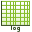# Changing the Axis Scale to Logarithmic

Modify a graph to make use of a logarithmic scale when the data is spread over large range. A logarithmic (log) scale allows you to view the data on a non-linear scale.

A log scale can be applied to a Cartesian graph for both the horizontal and vertical axes. For a polar graph, a log scale can only be applied to the radial axis.
As an example, the horizontal axis of a Cartesian graph is changed to a log scale. The steps are similar for changing the vertical axis of a Cartesian graph or the radial axis of a polar graph.
1. Select the Cartesian graph where you want to enable the log scaling for the horizontal axis.
2. On the Display tab, in the Axes group, click theLog (horizontal) icon.